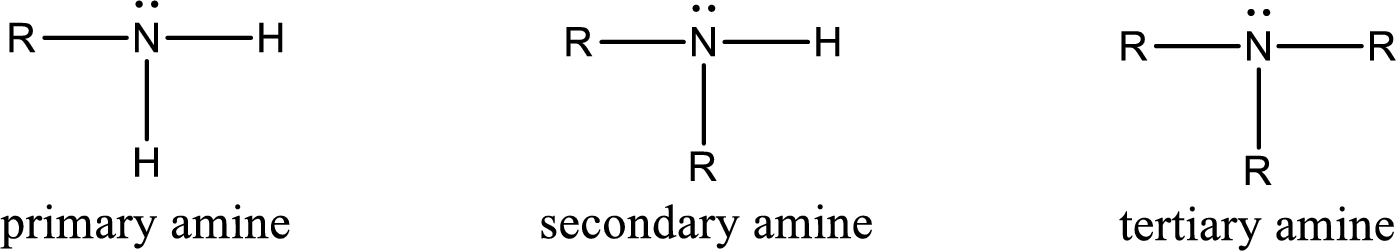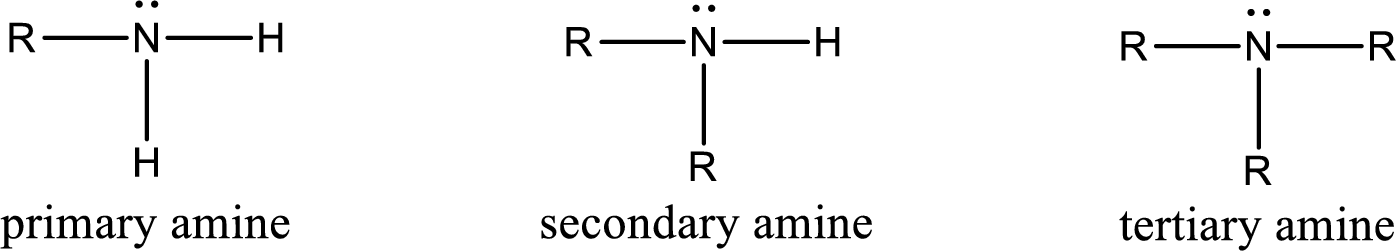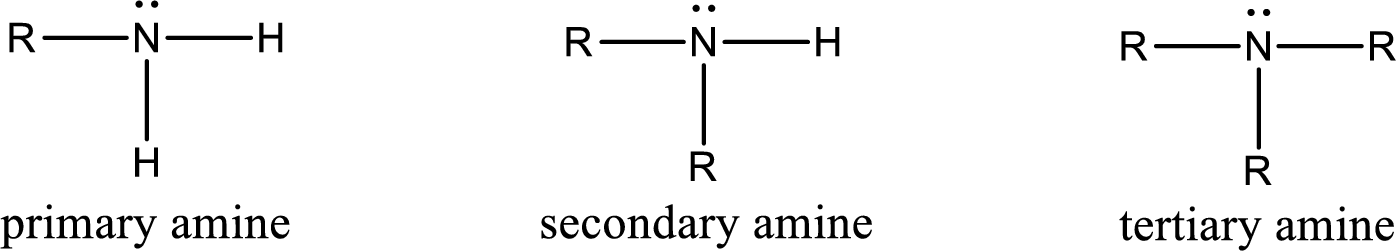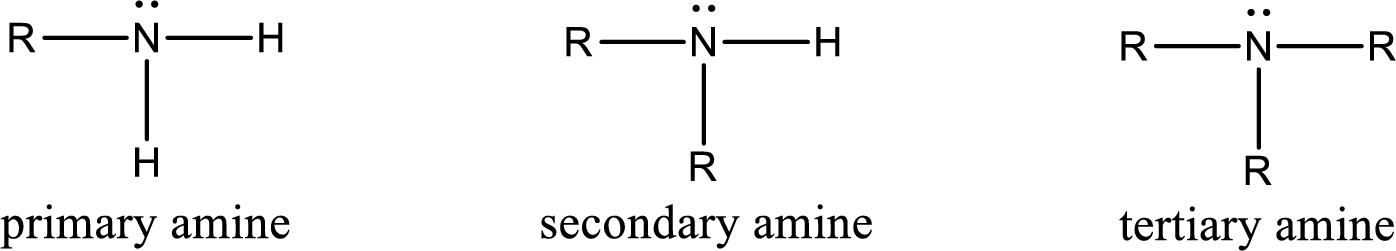Chapter 6, Problem 6.65EP### Organic And Biological Chemistry

7th Edition
STOKER + 1 other
ISBN: 9781305081079

#### Solutions

Chapter
Section### Organic And Biological Chemistry

7th Edition
STOKER + 1 other
ISBN: 9781305081079
Textbook Problem

# For each of the reactions in Problem 17-61, draw the structure of the organic product and give the formulas of the two inorganic products produced. a. NH3 + CH3—CH2—CH2—Cl + NaOH →b. CH3—CH2—NH2 + CH3—CH2—Cl + NaOH →(a)

Interpretation Introduction

Interpretation:

The structure of organic product and formulas of the inorganic product formed in the given reaction has to be drawn.

Concept Introduction:

Alkylation reaction is a reaction in which the transfer of alkyl group from one molecule to another molecule takes place.  While considering amines, the alkylating agent that is used is alkyl halides.  Alkylation is done under basic conditions.  The general equations for amines alkylation process is,

Ammonia + Alkylhalide Base amineamine + Alkylhalide Base amineamine + Alkylhalide Base amineamine + Alkylhalide Base quaternary ammonium salt

Amine is an organic derivative.  If in ammonia one or more alkyl, cycloalkyl, or aryl groups are substituted instead of hydrogen atom then it is known as amine.  Depending on the number of substitution the amines are classified as primary, secondary or tertiary amine.  Primary amine is the one in which only one hydrogen atom in ammonia is replaced by a hydrocarbon group.  Secondary amine is the one in which only two hydrogen atoms in ammonia is replaced by a hydrocarbon group.  Tertiary amine is the one in which all three hydrogen atoms in ammonia is replaced by a hydrocarbon group.  The generalized structural formula for all the amines is,Quaternary ammonium salt is the one that has four carbon atoms attached to the nitrogen atom.  This is formed by the reaction of tertiary amine with alkyl halide in presence of a strong base.

Explanation

Given reaction is,

The reactants given in the above reaction are ammonia, propyl chloride.  Sodium hydroxide is a reagent that is used for basic condition in this case.  As the reaction between ammonia and propyl chloride gives propylamine as the product, this is an alkylation reaction...

(b)

Interpretation Introduction

Interpretation:

The structure of organic product and formulas of the inorganic product formed in the given reaction has to be drawn.

Concept Introduction:

Alkylation reaction is a reaction in which the transfer of alkyl group from one molecule to another molecule takes place.  While considering amines, the alkylating agent that is used is alkyl halides.  Alkylation is done under basic conditions.  The general equations for amines alkylation process is,

Ammonia + Alkylhalide Base amineamine + Alkylhalide Base amineamine + Alkylhalide Base amineamine + Alkylhalide Base quaternary ammonium salt

Amine is an organic derivative.  If in ammonia one or more alkyl, cycloalkyl, or aryl groups are substituted instead of hydrogen atom then it is known as amine.  Depending on the number of substitution the amines are classified as primary, secondary or tertiary amine.  Primary amine is the one in which only one hydrogen atom in ammonia is replaced by a hydrocarbon group.  Secondary amine is the one in which only two hydrogen atoms in ammonia is replaced by a hydrocarbon group.  Tertiary amine is the one in which all three hydrogen atoms in ammonia is replaced by a hydrocarbon group.  The generalized structural formula for all the amines is,Quaternary ammonium salt is the one that has four carbon atoms attached to the nitrogen atom.  This is formed by the reaction of tertiary amine with alkyl halide in presence of a strong base.

(c)

Interpretation Introduction

Interpretation:

The structure of organic product and formulas of the inorganic product formed in the given reaction has to be drawn.

Concept Introduction:

Alkylation reaction is a reaction in which the transfer of alkyl group from one molecule to another molecule takes place.  While considering amines, the alkylating agent that is used is alkyl halides.  Alkylation is done under basic conditions.  The general equations for amines alkylation process is,

Ammonia + Alkylhalide Base amineamine + Alkylhalide Base amineamine + Alkylhalide Base amineamine + Alkylhalide Base quaternary ammonium salt

Amine is an organic derivative.  If in ammonia one or more alkyl, cycloalkyl, or aryl groups are substituted instead of hydrogen atom then it is known as amine.  Depending on the number of substitution the amines are classified as primary, secondary or tertiary amine.  Primary amine is the one in which only one hydrogen atom in ammonia is replaced by a hydrocarbon group.  Secondary amine is the one in which only two hydrogen atoms in ammonia is replaced by a hydrocarbon group.  Tertiary amine is the one in which all three hydrogen atoms in ammonia is replaced by a hydrocarbon group.  The generalized structural formula for all the amines is,Quaternary ammonium salt is the one that has four carbon atoms attached to the nitrogen atom.  This is formed by the reaction of tertiary amine with alkyl halide in presence of a strong base.

(d)

Interpretation Introduction

Interpretation:

The structure of organic product and formulas of the inorganic product formed in the given reaction has to be drawn.

Concept Introduction:

Alkylation reaction is a reaction in which the transfer of alkyl group from one molecule to another molecule takes place.  While considering amines, the alkylating agent that is used is alkyl halides.  Alkylation is done under basic conditions.  The general equations for amines alkylation process is,

Ammonia + Alkylhalide Base amineamine + Alkylhalide Base amineamine + Alkylhalide Base amineamine + Alkylhalide Base quaternary ammonium salt

Amine is an organic derivative.  If in ammonia one or more alkyl, cycloalkyl, or aryl groups are substituted instead of hydrogen atom then it is known as amine.  Depending on the number of substitution the amines are classified as primary, secondary or tertiary amine.  Primary amine is the one in which only one hydrogen atom in ammonia is replaced by a hydrocarbon group.  Secondary amine is the one in which only two hydrogen atoms in ammonia is replaced by a hydrocarbon group.  Tertiary amine is the one in which all three hydrogen atoms in ammonia is replaced by a hydrocarbon group.  The generalized structural formula for all the amines is,Quaternary ammonium salt is the one that has four carbon atoms attached to the nitrogen atom.  This is formed by the reaction of tertiary amine with alkyl halide in presence of a strong base.

### Still sussing out bartleby?

Check out a sample textbook solution.

See a sample solution

#### The Solution to Your Study Problems

Bartleby provides explanations to thousands of textbook problems written by our experts, many with advanced degrees!

Get Started